# Discrete Mathematics and Combinatorics Commons™

1,116 Full-Text Articles 1,296 Authors 292,068 Downloads114 Institutions

## All Articles in Discrete Mathematics and Combinatorics

1,116 full-text articles. Page 6 of 44.

Directed Graphs Of The Finite Tropical Semiring, 2021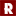Dordt University

#### Directed Graphs Of The Finite Tropical Semiring, Caden G. Zonnefeld

The focus of this paper lies at the intersection of the fields of tropical algebra and graph theory. In particular the interaction between tropical semirings and directed graphs is investigated. Originally studied by Lipvoski, the directed graph of a ring is useful in identifying properties within the algebraic structure of a ring. This work builds off research completed by Beyer and Fields, Hausken and Skinner, and Ang and Shulte in constructing directed graphs from rings. However, we will investigate the relationship (x, y)→(min(x, y), x+y) as defined by the operations of tropical algebra and applied to tropical semirings.

2021Institute of Mathematics Pedagogical University of Krakow

#### The Structure Of Functional Graphs For Functions From A Finite Domain To Itself For Which A Half Iterate Exists, Paweł Marcin Kozyra

##### Theory and Applications of Graphs

The notion of a replica of a nontrivial in-tree is defined. A result enabling to determine whether an in-tree is a replica of another in-tree employing an injective mapping between some subsets of sources of these in-trees is presented. There are given necessary and sufficient conditions for the existence of a functional square root of a function from a finite set to itself through presenting necessary and sufficient conditions for the existence of a square root of a component of the functional graph for the function and for the existence of a square root of the union of two components …

2021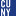CUNY La Guardia Community College

#### The “Knapsack Problem” Workbook: An Exploration Of Topics In Computer Science, Steven Cosares

##### Open Educational Resources

This workbook provides discussions, programming assignments, projects, and class exercises revolving around the “Knapsack Problem” (KP), which is widely a recognized model that is taught within a typical Computer Science curriculum. Throughout these discussions, we use KP to introduce or review topics found in courses covering topics in Discrete Mathematics, Mathematical Programming, Data Structures, Algorithms, Computational Complexity, etc. Because of the broad range of subjects discussed, this workbook and the accompanying spreadsheet files might be used as part of some CS capstone experience. Otherwise, we recommend that individual sections be used, as needed, for exercises relevant to a course in …

Determinant Formulas Of Some Hessenberg Matrices With Jacobsthal Entries, 2021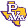Vasyl Stefanyk Precarpathian National University

#### Determinant Formulas Of Some Hessenberg Matrices With Jacobsthal Entries, Taras Goy, Mark Shattuck

##### Applications and Applied Mathematics: An International Journal (AAM)

In this paper, we evaluate determinants of several families of Hessenberg matrices having various subsequences of the Jacobsthal sequence as their nonzero entries. These identities may be written equivalently as formulas for certain linearly recurrent sequences expressed in terms of sums of products of Jacobsthal numbers with multinomial coefficients. Among the sequences that arise in this way include the Mersenne, Lucas and Jacobsthal-Lucas numbers as well as the squares of the Jacobsthal and Mersenne sequences. These results are extended to Hessenberg determinants involving sequences that are derived from two general families of linear second-order recurrences. Finally, combinatorial proofs are provided …

2021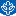DePaul University

#### Spring 2021

##### Scientia

From the Dean: A Decade of Purpose and Progress; Lab Notes: Alumna Wins Gordon Bell Special Prize, New Scholarships, Vaccination Site Volunteers; Women in Science Lecture, National Institutes of Health Grants, "Unequal Cities" Research; All Hands on Deck: Inspired pandemic approaches showcase interdisciplinary acumen in action; Unlocking Potential: Christopher Beasley thinks psychology is key to academic transformation for the formerly incarcerated; Puzzle Master: Bridget Tenner goes to pieces solving problems in cutting-edge mathematics

From Multi-Prime To Subset Labelings Of Graphs, 2021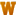Western Michigan University

#### From Multi-Prime To Subset Labelings Of Graphs, Bethel I. Mcgrew

##### Dissertations

A graph labeling is an assignment of labels (elements of some set) to the vertices or edges (or both) of a graph G. If only the vertices of G are labeled, then the resulting graph is a vertex-labeled graph. If only the edges are labeled, the resulting graph is an edge-labeled graph. The concept was first introduced in the 19th century when Arthur Cayley established Cayley’s Tree Formula, which proved that there are nn-2 distinct labeled trees of order n. Since then, it has grown into a popular research area.

In this study, we first review several types …

On The Hamiltonicity Of Subgroup Lattices, 2021Missouri State University

#### On The Hamiltonicity Of Subgroup Lattices, Nicholas Charles Fleece

In this paper we discuss the Hamiltonicity of the subgroup lattices of

different classes of groups. We provide sufficient conditions for the

Hamiltonicity of the subgroup lattices of cube-free abelian groups. We also

prove the non-Hamiltonicity of the subgroup lattices of dihedral and

dicyclic groups. We disprove a conjecture on non-abelian p-groups by

producing an infinite family of non-abelian p-groups with Hamiltonian

subgroup lattices. Finally, we provide a list of the Hamiltonicity of the

subgroup lattices of every finite group up to order 35 barring two groups.

Classification Of Cayley Rose Window Graphs, 2021Presidency University, Kolkata

#### Classification Of Cayley Rose Window Graphs, Angsuman Das, Arnab Mandal

##### Theory and Applications of Graphs

Rose window graphs are a family of tetravalent graphs, introduced by Steve Wilson. Following it, Kovacs, Kutnar and Marusic classified the edge-transitive rose window graphs and Dobson, Kovacs and Miklavic characterized the vertex transitive rose window graphs. In this paper, we classify the Cayley rose window graphs.

2021Department of Mathematics, Faculty of Science, University of Malta, Malta

#### Reducing The Maximum Degree Of A Graph: Comparisons Of Bounds, Peter Borg

##### Theory and Applications of Graphs

Let $\lambda(G)$ be the smallest number of vertices that can be removed from a non-empty graph $G$ so that the resulting graph has a smaller maximum degree. Let $\lambda_{\rm e}(G)$ be the smallest number of edges that can be removed from $G$ for the same purpose. Let $k$ be the maximum degree of $G$, let $t$ be the number of vertices of degree $k$, let $M(G)$ be the set of vertices of degree $k$, let $n$ be the number of vertices in the closed neighbourhood of $M(G)$, and let $m$ be the number of edges that have at least one …

Compact Representations Of Uncertainty In Clustering, 2021University of Massachusetts Amherst

#### Compact Representations Of Uncertainty In Clustering, Craig Stuart Greenberg

##### Doctoral Dissertations

Flat clustering and hierarchical clustering are two fundamental tasks, often used to discover meaningful structures in data, such as subtypes of cancer, phylogenetic relationships, taxonomies of concepts, and cascades of particle decays in particle physics. When multiple clusterings of the data are possible, it is useful to represent uncertainty in clustering through various probabilistic quantities, such as the distribution over partitions or tree structures, and the marginal probabilities of subpartitions or subtrees.

Many compact representations exist for structured prediction problems, enabling the efficient computation of probability distributions, e.g., a trellis structure and corresponding Forward-Backward algorithm for Markov models that model …

Emergent Hierarchy Through Conductance-Based Degree Constraints, 2021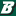Air Force Research Laboratory / Clarkson University

#### Emergent Hierarchy Through Conductance-Based Degree Constraints, Christopher Tyler Diggans, Jeremie Fish, Erik M. Bollt

##### Northeast Journal of Complex Systems (NEJCS)

The presence of hierarchy in many real-world networks is not yet fully understood. We observe that complex interaction networks are often coarse-grain models of vast modular networks, where tightly connected subgraphs are agglomerated into nodes for simplicity of representation and computational feasibility. The emergence of hierarchy in such growing complex networks may stem from one particular property of these ignored subgraphs: their graph conductance. Being a quantification of the main bottleneck of flow through the coarse-grain node, this scalar quantity implies a structural limitation and supports the consideration of heterogeneous degree constraints. The internal conductance values of the subgraphs are …

2021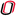University of Nebraska at Omaha

#### Optimizing Networking Topologies With Shortest Path Algorithms, Jordan Sahs

##### UNO Student Research and Creative Activity Fair

Communication networks tend to contain redundant devices and mediums of transmission, thus the need to locate, document, and optimize networks is increasingly becoming necessary. However, many people do not know where to start the optimization progress. What is network topology? What is this “Shortest Path Problem”, and how can it be used to better my network? These questions are presented, taught, and answered within this paper. To supplement the reader’s understanding there are thirty-eight figures in the paper that are used to help convey and compartmentalize the learning process needed to grasp the materials presented in the ending sections.

In …

The Conditional Strong Matching Preclusion Of Augmented Cubes, 2021American University of Kuwait

#### The Conditional Strong Matching Preclusion Of Augmented Cubes, Mohamad Abdallah, Eddie Cheng

##### Theory and Applications of Graphs

The strong matching preclusion is a measure for the robustness of interconnection networks in the presence of node and/or link failures. However, in the case of random link and/or node failures, it is unlikely to find all the faults incident and/or adjacent to the same vertex. This motivates Park et al. to introduce the conditional strong matching preclusion of a graph. In this paper we consider the conditional strong matching preclusion problem of the augmented cube $AQ_n$, which is a variation of the hypercube $Q_n$ that possesses favorable properties.

2021Wright State University

#### Characterizing 2-Trees Relative To Chordal And Series-Parallel Graphs, Terry A. Mckee

##### Theory and Applications of Graphs

The 2-connected 2-tree graphs are defined as being constructible from a single 3-cycle by recursively appending new degree-2 vertices so as to form 3-cycles that have unique edges in common with the existing graph. Such 2-trees can be characterized both as the edge-minimal chordal graphs and also as the edge-maximal series-parallel graphs. These are also precisely the 2-connected graphs that are simultaneously chordal and series-parallel, where these latter two better-known types of graphs have themselves been both characterized and applied in numerous ways that are unmotivated by their interaction with 2-trees and with each other.

Toward providing such motivation, the …

2021Karshi State University

#### Innovative Approach To Solving Combinatic Elements And Some Problems Of Newton Binomy In School Mathematics Course, Nilufar Okbayeva

##### Central Asian Problems of Modern Science and Education

This article provides information on the elements of combinatorics in the school mathematics course and solutions to some problems related to the Newtonian binomial. This article is also aimed at solving problems related to the indepth study of the elements of combinatorics in the school course, the creation of a sufficient basis for the study of probability theory and mathematical statistics in the future.

Skolem Number Of Subgraphs On The Triangular Lattice, 2021Southern Connecticut State University

#### Skolem Number Of Subgraphs On The Triangular Lattice, Braxton Carrigan, Garrett Green

##### Communications on Number Theory and Combinatorial Theory

A Skolem sequence can be thought of as a labelled path where any two vertices with the same label are that distance apart. This concept has naturally been generalized to graph labelling. This brings rise to the question; “what is the smallest set of consecutive positive integers we can use to properly Skolem label a graph?” This is known as the Skolem number of the graph. In this paper we give the Skolem number for three natural vertex induced subgraphs of the triangular lattice graph.

Connectivity Of Matroids And Polymatroids, 2021Louisiana State University and Agricultural and Mechanical College

#### Connectivity Of Matroids And Polymatroids, Zachary R. Gershkoff

##### LSU Doctoral Dissertations

This dissertation is a collection of work on matroid and polymatroid connectivity. Connectivity is a useful property of matroids that allows a matroid to be decomposed naturally into its connected components, which are like blocks in a graph. The Cunningham-Edmonds tree decomposition further gives a way to decompose matroids into 3-connected minors. Much of the research below concerns alternate senses in which matroids and polymatroids can be connected. After a brief introduction to matroid theory in Chapter 1, the main results of this dissertation are given in Chapters 2 and 3. Tutte proved that, for an element e of a …

Determining Biases In The Card-Chameleon Cryptosystem, 2021Kutztown University of Pennsylvania

#### Determining Biases In The Card-Chameleon Cryptosystem, Isaac Reiter, Eric Landquist

##### Communications on Number Theory and Combinatorial Theory

Throughout history, spies, soldiers, and others have relied on so-called {\em hand ciphers} to send encrypted messages. Since the creation of Pontifex (also known as Solitaire) by Bruce Schneier in 1999, a number of hand ciphers utilizing a standard deck of playing cards have emerged. Since there are $52! \approx 2^{225.58}$ possible ways to order a deck of cards, there are over 225 bits of entropy in a well-shuffled deck of cards. Theoretically, this can provide enough security to rival modern computer-based cryptosystems. In this paper, we describe and analyze one such playing card cipher, Card-Chameleon, created by Matthew McKague. …

2021Georgia Southern University

#### An Efficient Algorithm To Test Potential Bipartiteness Of Graphical Degree Sequences, Kai Wang

##### Theory and Applications of Graphs

As a partial answer to a question of Rao, a deterministic and customizable efficient algorithm is presented to test whether an arbitrary graphical degree sequence has a bipartite realization. The algorithm can be configured to run in polynomial time, at the expense of possibly producing an erroneous output on some yes'' instances but with very low error rate.

Nilpotent Graph, 2021Tezpur University, India

#### Nilpotent Graph, Dhiren Kumar Basnet, Ajay Sharma, Rahul Dutta

##### Theory and Applications of Graphs

In this article, we introduce the concept of nilpotent graph of a finite commutative ring. The set of all non nilpotent elements of a ring is taken as the vertex set and two vertices are adjacent if and only if their sum is nilpotent. We discuss some graph theoretic properties of nilpotent graph.